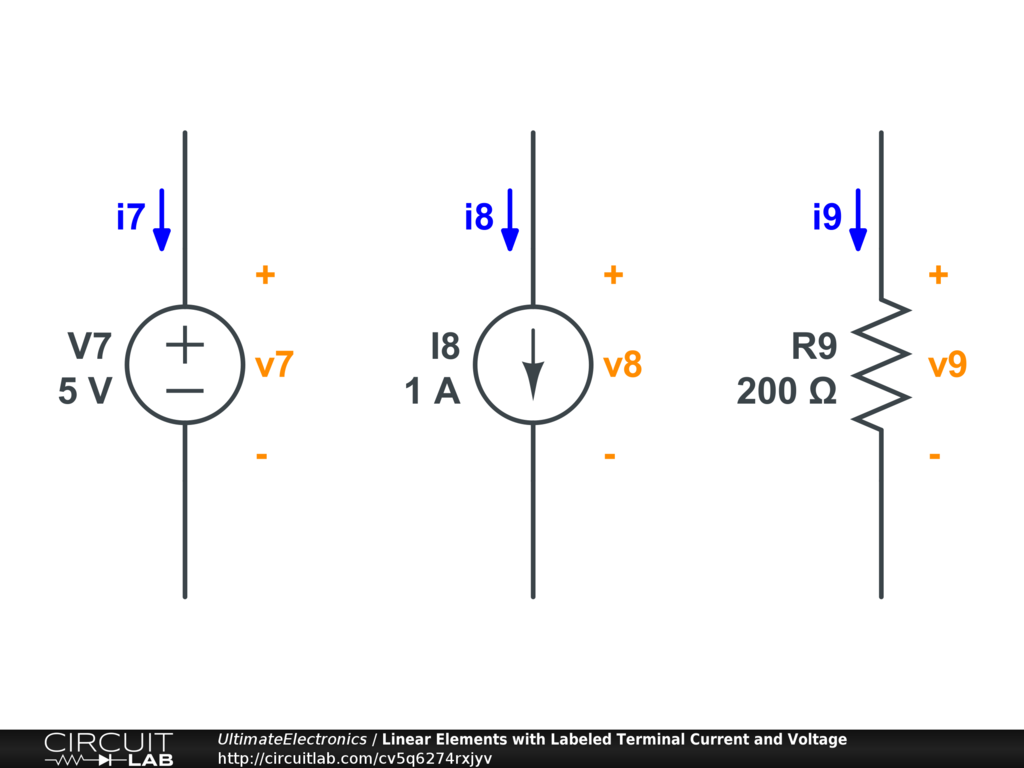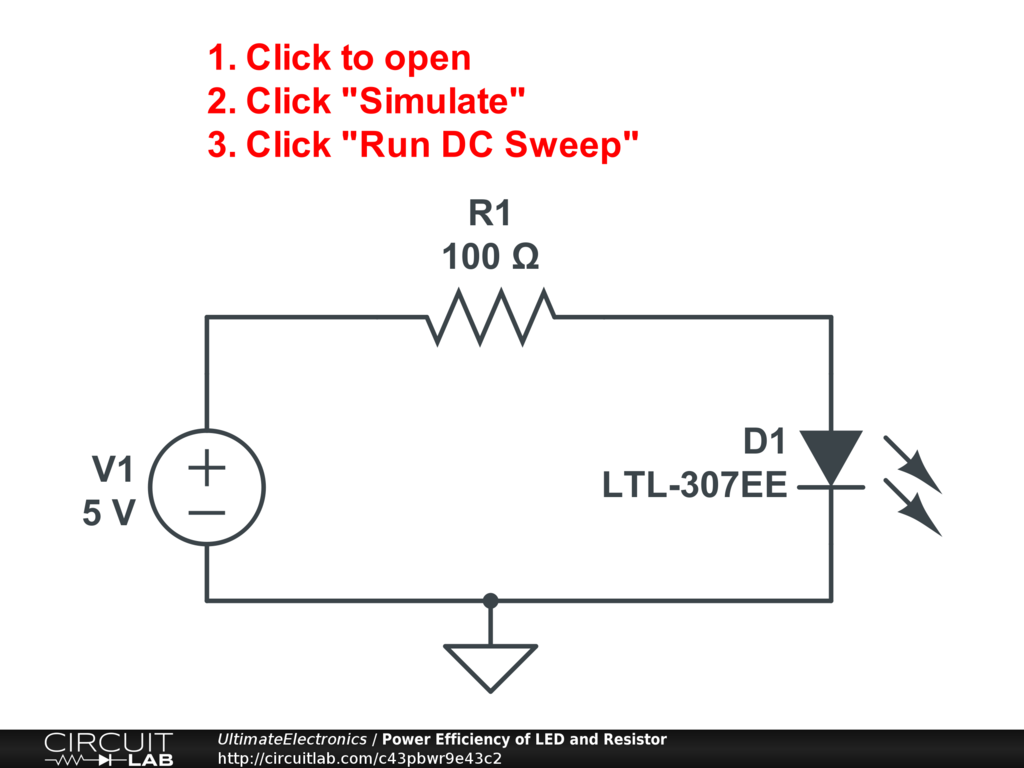Power

Power is the rate of flow of energy:

$$\text{Power} = \frac{\text{Energy}} {\text{Time}}$$

Energy was previously discussed in the Thermodynamics, Energy, and Equilibrium section.

Power has units of Watts.

\begin{align} 1 \ \text{Watt} & = 1 \ \frac {\text{Joule}} {\text{second}} \\ 1 \ \text{W} & = 1 \ \frac {\text{J}} {\text{s}} \end{align}

In the Lumped Element Model, power can flow either into an element, or out of an element. The sign of power indicates the direction of energy flow.

The net power on the schematic of a closed system is zero. This is the First Law of Thermodynamics in action. Energy is conserved: it is neither created nor destroyed. (See below for some potential gotchas when applying this rule to a schematic!)

In general, we can choose the signs arbitrarily, but we'll define that a source has negative power (i.e. it is supplying power to the schematic), and a load has positive power (i.e. it is consuming power from the schematic). We'll define it this way by being careful about how we define our voltage and current directions.

Labeling the direction of current and voltage at each terminal properly is critically important for solving circuits. We'll talk more about this in a future section Labeling Voltages, Currents, and Nodes after we study Kirchhoff's Laws.

Two-Terminal Element Power

For a circuit element with only two terminals, we can write the power $P$ as:

$$P = v i$$

where $v$ is the voltage difference between the two terminals, and $i$ is the current. (The same value of current must be going into one of the terminals and out of the other.)

Consider these three two-terminal elements. We've labeled a positive and negative end for each voltage difference. By convention, we must define the current as going into the positive terminal. (Otherwise, we would have to write $P = - v i$.)As labeled, the power consumed by each element will be:

\begin{align} P_{V7} & = v_7 i_7 \\ P_{I8} & = v_8 i_8 \\ P_{R9} & = v_9 i_9 \end{align}

If that element is consuming power, the value will be positive.

If the element is supplying power, the value will be negative.

Off-Schematic Energy

If a resistor is consuming power, that power is being turned into heat. That heat might be building up within the resistor (causing its temeperature to increase), or it might be dissipated to the surroundings by conduction, convection, or radiation. (See Practical Resistors: Power Rating (Wattage) for more.)

If a voltage source is supplying power, that power is coming from somewhere else, such as from an electrochemical reaction in a battery.

A capacitor while charging will be consuming power from the schematic. This energy will be stored in the electric field within the dielectric material of the capacitor. When the capacitor is discharging, the capacitor will be supplying power to other elements on the schematic.

In a double-entry bookkeeping sense, the circuit network only represents one side of the energy transaction of each component. There is an equal and opposite side of that energy flow, but what it is depends on the specific element and is not necessarily represented on the schematic.

Multi-Terminal Element Power

More generally, for a multi-terminal component with two or more terminals:

$$P = \sum_{t} v_t i_t$$

At each terminal, we multiply the terminal voltage (with respect to ground) by the current into that terminal.

The choice of ground does not matter because we can add any number arbitrarily to all $v_t$ values without changing $P$:

$$P = \sum_{t} v_t i_t = \sum_{t} (v_t - v_\text{ref}) i_t \quad \text{for any} \quad v_{\text{ref}}$$

because:

$$\sum_{t} v_{\text{ref}} i_t = v_{\text{ref}} \sum_{t} i_t = v_{\text{ref}} \cdot 0 = 0$$

The fact that $\sum_{t} i_t = 0$ is one way to express Kirchhoff's Current Law. All currents flowing into any device must sum to zero because no net charge can accumulate within the device.

You can use this summation rule for three-terminal devices such as transistors, or devices with any number of terminals you'd like. However, be aware that some components like op-amps and digital logic gates are often represented on the schematic with some terminals hidden from view.

In the two-terminal case, this multi-terminal rule just becomes the same as the simplified rule above. This is because $v = v_1 - v_2$ (by definition of voltage difference across the two terminals) and $i = i_1 = -i_2$ (because the current flowing into one terminal must flow out of the other), so:

\begin{align} P & = v_1 i_1 + v_2 i_2 \\ P & = v_1 i + v_2 (-i) \\ P & = (v_1 - v_2) i \\ P & = v i \end{align}

Power in Resistors

For a linear resistor obeying Ohm's Law:

$$P = v i = i^2 R = \frac{v^2}{R}$$

In problem solving, all three forms of this power dissipation are useful.

The resistor turns electrical energy into heat via inelastic collisions in the resistive material.

If current is known, use the $P = i^2 R$ form.

If voltage is known, use the $P = \frac{v^2}{R}$ form.

Efficiency

Efficiency was previously discussed in the Thermodynamics, Energy, and Equilibrium section.

We have to define our efficiency in each particular application, but it always is a ratio of powers or a ratio of energies.

For a quick example, though we haven't talked about LEDs yet, let's consider the efficiency of driving an LED from voltage source with a series resistor.

Imagine that this is a battery powered flashlight, and we're concerned about how much power goes into the LED versus how much is wasted as heat in the resistor. In that case, we could define our efficiency as:

\begin{align} \eta & = \frac { \text{Power consumed by LED} } { \text{Power supplied by source} } \\ & = \frac {P_{\text{D1}}} {-P_{\text{V1}}} \end{align}

Here's a schematic:Interactive Exercise Click the circuit, then click "Simulate," and "Run DC Sweep." You'll see a plot of the expression "P(D1)/-P(V1)", which is the efficiency $\eta$ we defined above expressed in CircuitLab's expression notation.

Observe that for different input voltages, the efficiency changes. And overall, the efficiency when driving from a 5V voltage source is only around 43%. We're losing a lot of our battery energy just to heat up a resistor!

This is only the electrical efficiency; there is still the quantum efficiency of the LED which we may wish to take into account, because not all power delivered to the LED is turned into light. Some is turned into heat within the LED.

If you'd like to play around with this circuit at this point, you're encouraged to.

• What happens if you change the resistance?
• How much light is going to be emitted -- assuming the amount of light is proportional to the current through the LED?
• What if the LED will overheat if it has to dissipate over 50 mW?

Energy

Energy is measured in Joules:

$$E = P t$$

We pay the electric company for energy delivered. Joules can be converted to kilowatt hours through a simple unit conversion:

$$1 \ \text{kWh} = 3.6 \times 10^6 \ \text{J}$$

Batteries store energy, though often we talk about them in terms of amp-hours (current-times-time rather than power-times-time).

Really, energy is an integral of a possibly time-varying power:

$$E = \int_{t_1}^{t_2} P(t) dt$$

In many practical systems, power will not be steady, but will vary substantially over time. This integral takes care of that by looking at total energy, rather than instantaneous power.

Refer to the Schematics & Simulations Tutorial to see how to compute integrals in CircuitLab's time-domain simulation plotting window.

Instantaneous verus Average Power

As electrical power is transformed to and from other forms of energy, there are often other time constants at work.

For example, when we turn electrical energy into light in an LED, we may turn the light on and off very fast -- faster than the human eye can discern. Or, when we control a motor via PWM, we may supply and interrupt power to the motor very very fast -- but the mechanical inertia of whatever's being driven hides this fact.

When we design electrical or other systems, it is critical that we care about both the instantaneous power and the average power. As discussed in the Steady State & Transient section, the physical stresses on the various components of our system can be substantially higher with respect to instantaneous or transient behavior, and we have to design everything to accomodate those forces.

Imagine that we have a spaceship powered by dropping a thermonuclear bomb out of the back and detonating it to push the ship forward once every 60 seconds. In this case, we have to design the spaceship to be able to tolerate the instaneous blast force of the explosion, and the total integrated impulse provided by these blasts must provide sufficient force to get the spaceship moving as fast as it needs to be for our desired trajectory! (As crazy as this sounds, it was seriously studied in the 1950s and 60s: 1 2 3.)

As an engineer, you must understand when either the instantaneous or average viewpoint matters, and design accordingly.

Power and Energy are Conserved

As a consequence of Kirchhoff's Voltage Law and Kirchhoff's Current Law, which we'll be discussing shortly, the power and energy on a schematic are conserved.

Energy may flow out of something, like a battery, which means that (outside of the schematic) that energy came from chemical potential energy.

Energy may flow into something, like a resistor, which means that (outside of the schematic) that energy became heat.

Or it may flow bidirectionally, for example, first into a capacitor, and then back out.

Just as net charge can't be stored "on the schematic," neither can net energy. The Lumped Element Model assumes that this is instantaneous: if one element is supplying power, others must be consuming the same amount simultaneously.

Sometimes, however, schematics are drawn for convenience in a way that hides the source of energy. For example, some op-amps and digital logic gates are drawn without their power rail connections. Or, a node can be labeled "+5" which implies that there's a voltage source somewhere, but it isn't drawn explicitly on the schematic. In these situations, don't forget to account for the implied additional edges of the circuit network.

What's Next

In the next section, Practical Resistors: Manufacturing Tolerances, Common Values, and Color Codes, we'll take a closer look at resistors and how they're manufactured and sold.